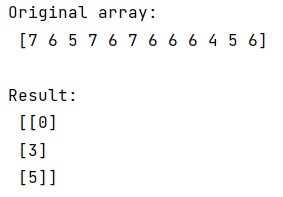# How to make numpy.argmax() return all occurrences of the maximum?

Learn, how to make numpy.argmax() return all occurrences of the maximum in Python?
Submitted by Pranit Sharma, on January 12, 2023

NumPy is an abbreviated form of Numerical Python. It is used for different types of scientific operations in python. Numpy is a vast library in python which is used for almost every kind of scientific or mathematical operation. It is itself an array which is a collection of various methods and functions for processing the arrays.

## Making numpy.argmax() return all occurrences of the maximum

Suppose that we are given a NumPy array that contains some integer value. The maximum of these values is present multiple times. To find the position of the maximum values in this NumPy array, we can simply use numpy.argmax() method. But this method will not return all the occurrences of the maximum value and hence we need to find another strategy to solve this problem.

An appropriate option is using np.argwhere in combination with np.amax. The numpy.argwhere() will return the positions of the element which satisfies the condition and numpy amax will return the maximum element. Hence, we will check where the maximum elements are present and return all the positions.

Let us understand with the help of an example,

## Python code to make numpy.argmax() return all occurrences of the maximum

```# Import numpy
import numpy as np

# Creating numpy array
arr = np.array([7, 6, 5, 7, 6, 7, 6, 6, 6, 4, 5, 6])

# Display original array
print("Original array:\n",arr,"\n")

# Return all the positions of max value
res = np.argwhere(arr == np.amax(arr))

# Display result
print("Result:\n",res)
```

Output:What's New (MCQs)

Top Interview Coding Problems/Challenges!

IncludeHelp's Blogs

Languages: » C » C++ » C++ STL » Java » Data Structure » C#.Net » Android » Kotlin » SQL
Web Technologies: » PHP » Python » JavaScript » CSS » Ajax » Node.js » Web programming/HTML
Solved programs: » C » C++ » DS » Java » C#
Aptitude que. & ans.: » C » C++ » Java » DBMS
Interview que. & ans.: » C » Embedded C » Java » SEO » HR
CS Subjects: » CS Basics » O.S. » Networks » DBMS » Embedded Systems » Cloud Computing
» Machine learning » CS Organizations » Linux » DOS
More: » Articles » Puzzles » News/Updates